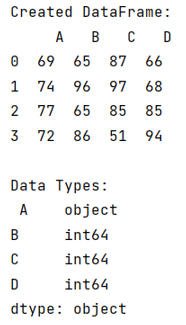# Import pandas DataFrame column as string not int

Learn, importing pandas DataFrame column as string not int. By Pranit Sharma Last updated : September 23, 2023

Pandas is a special tool that allows us to perform complex manipulations of data effectively and efficiently. Inside pandas, we mostly deal with a dataset in the form of DataFrame. DataFrames are 2-dimensional data structures in pandas. DataFrames consist of rows, columns, and data.

## Importing pandas DataFrame column as string not int

Sometimes we need to import a CSV file and we want the columns as strings, not int, for this purpose while importing the CSV file, we define a dictionary inside the read_csv() method. This dictionary consists of a key that has the column name and the values which have their dtype.

Note

To work with pandas, we need to import pandas package first, below is the syntax:

```import pandas as pd
```

Let us understand with the help of an example,

## Python program to import pandas DataFrame column as string not int

```# Importing pandas package
import pandas as pd

# Importing a csv file

# Creating a DataFrame
df = pd.DataFrame(data)

# Display DataFrame
print("Created DataFrame:\n",df,"\n")

# Check the dtype of each colum
print("Data Types:\n",df.dtypes)
```

### Output

The output of the above program is: